Question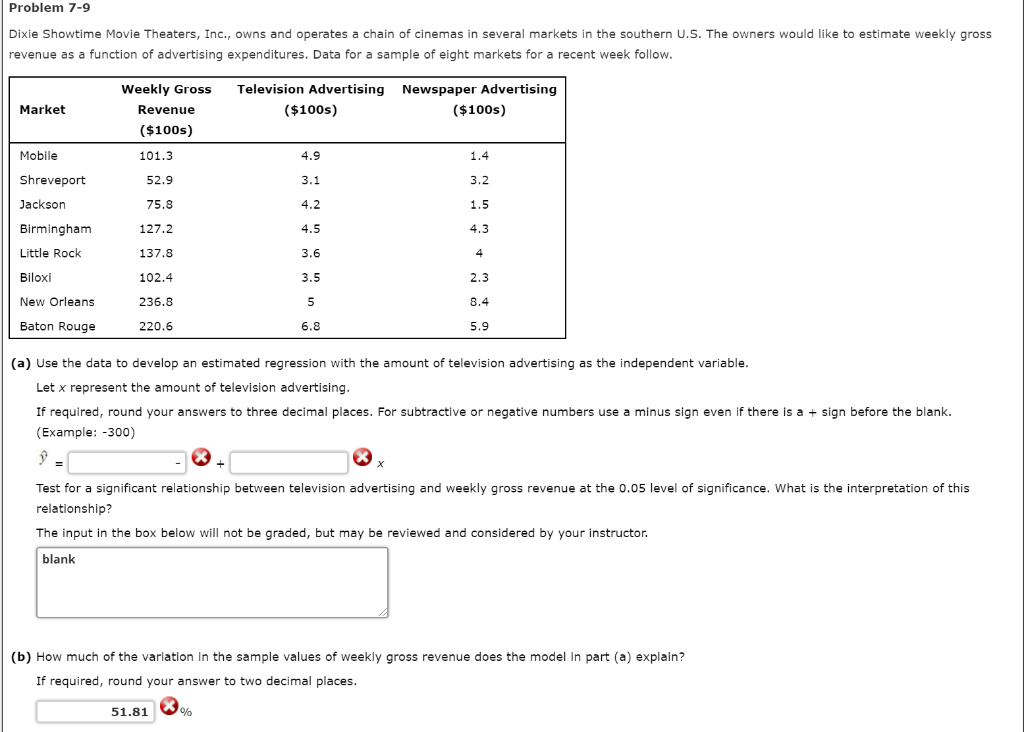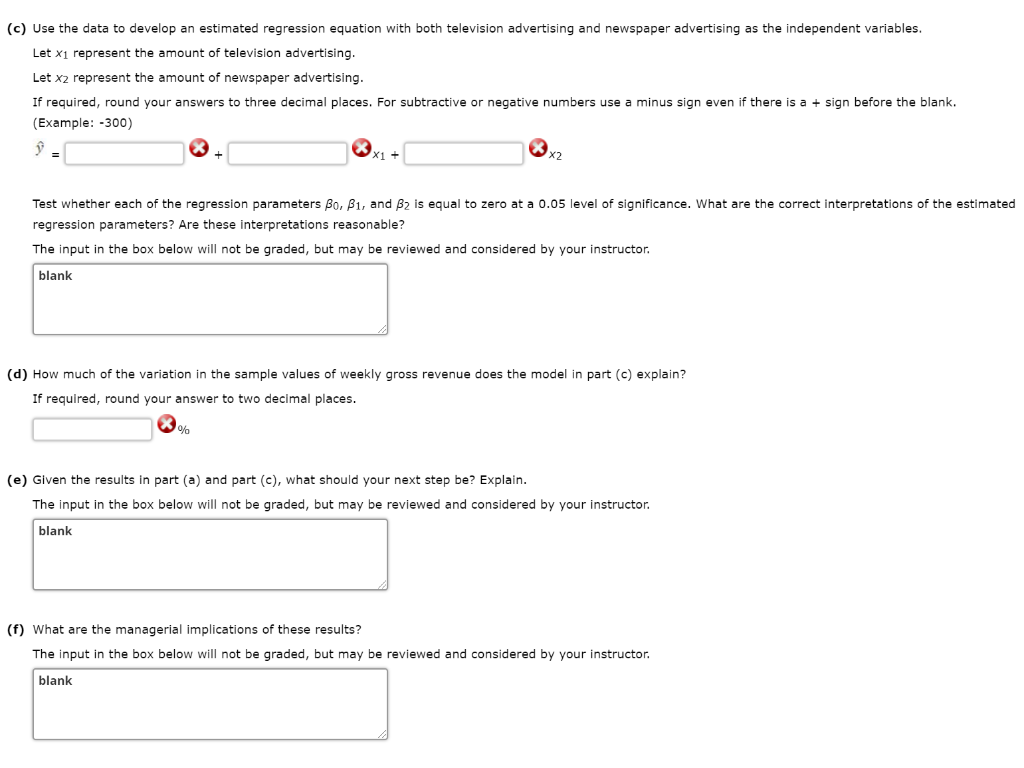In order to solve this question I used R software.

R codes and output:

Revenue TV Newspaper

1   101.3 4.9       1.4

2    52.9 3.1       3.2

3    75.8 4.2       1.5

4   127.2 4.5       4.3

5   137.8 3.6       4.0

6   102.4 3.5       2.3

> attach(d)

> fit=lm(Revenue ~ TV)

> summary(fit)

Call:

lm(formula = Revenue ~ TV)

Residuals:

Min      1Q Median      3Q     Max

-49.221 -28.623 -7.739 17.779 82.130

Coefficients:

Estimate Std. Error t value Pr(>|t|)

(Intercept)   -52.783 70.89 -0.745   0.4847

TV             41.491 15.47   2.682   0.0364 *

---

Signif. codes: 0 ‘***’ 0.001 ‘**’ 0.01 ‘*’ 0.05 ‘.’ 0.1 ‘ ’ 1

Residual standard error: 47.79 on 6 degrees of freedom

Multiple R-squared: 0.5452, Adjusted R-squared: 0.4694

F-statistic: 7.192 on 1 and 6 DF, p-value: 0.03645

> fit2=lm(Revenue ~ TV + Newspaper)

> summary(fit2)

Call:

lm(formula = Revenue ~ TV + Newspaper)

Residuals:

1       2       3       4       5       6       7       8

5.858 -34.433 -5.100 -13.891 23.540 22.755   6.147 -4.875

Coefficients:

Estimate Std. Error t value Pr(>|t|)

(Intercept) -46.208     33.678 -1.372 0.22841

TV            23.485      8.302   2.829 0.03672 *

Newspaper     18.980      4.081   4.651 0.00558 **

---

Signif. codes: 0 ‘***’ 0.001 ‘**’ 0.01 ‘*’ 0.05 ‘.’ 0.1 ‘ ’ 1

Residual standard error: 22.68 on 5 degrees of freedom

Multiple R-squared: 0.9146, Adjusted R-squared: 0.8804

F-statistic: 26.78 on 2 and 5 DF, p-value: 0.002131

a.

y = -52.783 + 41.491 x

Since p-value for testing slope coefficient of TV is 0.0364, which is less than 0.05, hence we conclude that Slope coefficient is statistically significant. Which ultimately implies that there is significant relationship between television advertising and weekly gross revenue.

b.

54.52% variation.

c.

y =  -46.208 + 23.485 x1 + 18.980 x2

P-value for intercept is greater than 0.05, hence intercept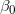is not statistically significant. P-value for slope coefficient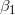and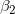are less that 0.05, hence these variables are statistically significant.

d.

91.46% variation.

e.

R2 and adjusted R2 both are high for second model, it means second model will explain more variation in the weekly gross revenue. Hence we choose second model for prediction.

f.

Manager would prefer model 2.

#### Earn Coins

Coins can be redeemed for fabulous gifts.

Similar Homework Help Questions
• ### 12.3 A statistical program is recommended. The owner of Showtime Movie Theaters, Inc., would like to...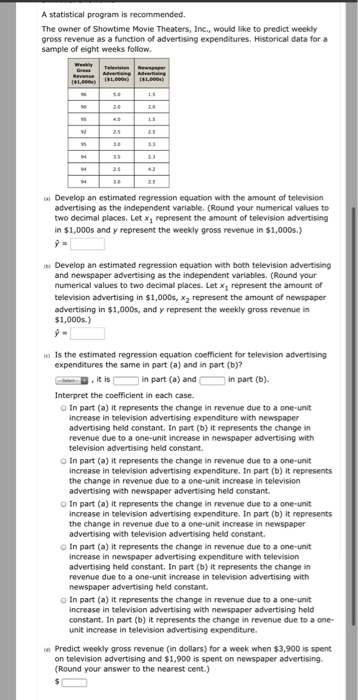12.3 A statistical program is recommended. The owner of Showtime Movie Theaters, Inc., would like to predict weekly gross revenue as a function of advertising expenditures. Historical data for a sample of eight weeks follow Develop an estimated regression equation with the amount of television advertising as the independent variable. (Round your numerical values to two decimal places. Let x, represent the amount of television advertising in $1,000s and y represent the weekly gross revenue in$1,000s.) Develop an estimated...

• ### Question 1The owner of Showtime Movie Theaters, Inc. would like to predict weekly gross revenue as...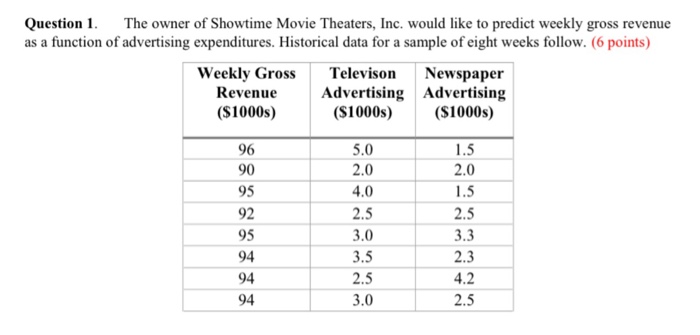Question 1The owner of Showtime Movie Theaters, Inc. would like to predict weekly gross revenue as a function of advertising expenditures. Historical data for a sample of eight weeks follow. (6 points) Weekly Gross Revenue Newspaper Advertising Advertising ($1000s) Televison ($1000s) (s1000s) 96 5.0 1.5 2.0 2.0 90 95 4.0 1.5 92 2.5 2.5 3.3 95 3.0 3.5 2.3 94 2.5 4.2 94 94 3.0 2.5 b. Develop an estimated regression equation with both television advertising and news- paper advertising...

• ### Question 1The owner of Showtime Movie Theaters, Inc. would like to predict weekly gross revenue as...Question 1The owner of Showtime Movie Theaters, Inc. would like to predict weekly gross revenue as a function of advertising expenditures. Historical data for a sample of eight weeks follow. (6 points) Weekly Gross Revenue Newspaper Advertising Advertising ($1000s) Televison ($1000s) (s1000s) 96 5.0 1.5 2.0 2.0 90 95 4.0 1.5 92 2.5 2.5 3.3 95 3.0 3.5 2.3 94 2.5 4.2 94 94 3.0 2.5 Question 2: In Question 1, the owner of Showtime Movie Theaters, Inc. used multiple...